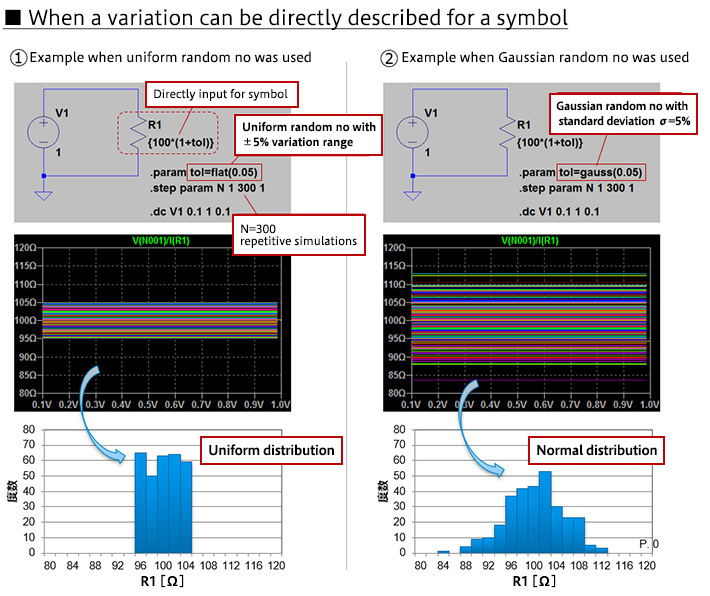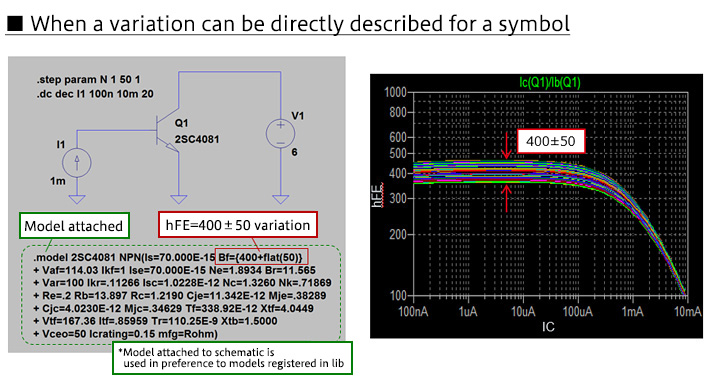Technical Information Site of Power Supply Design

2018.12.20 Simulation

# Types of SPICE simulation: Monte Carlo

Foundations of Electronic Circuit Simulation

In the previous article we described SPICE simulation types and explained DC analysis, AC analysis, and transient analysis among the four types of analysis functions provided as standard. In this article, we explain the remaining function, Monte Carlo simulations.

SPICE simulation: Monte Carlo

Monte Carlo is a general term for methods using random numbers in simulations and numerical calculations. Nearly all the components used to create actual circuits--resistors, capacitors, inductors, diodes, transistors, ICs, and so on--have characteristics with variations. For example, a resistor has a tolerance: the resistance value of a resistor that is nominally 100 Ω with a precision of ±5% is between 95 Ω and 105 Ω. There is variation in each individual component, and the sum of the variations of the components results in variation in the characteristics of the circuit.

In a Monte Carlo simulation, simulations that reflect the variation in the different circuit elements are executed a number of times, and variation in the overall characteristics can be evaluated. This is effective when the characteristics of the overall circuit are affected by multiple circuit elements. Various methods are used, but in essence the variations mainly of the circuit elements that have the largest effect on the overall circuits are added.

Monte Carlo Example

Methods for setting variations differ depending on the simulation type. Monte Carlo setting methods are also different depending on whether variations can or cannot be directly stated for resistors and other symbols. Here examples of each are given.

・Case in which variations can be directly described for symbols

In this example, a simulation is performed of the resistance value (voltage/current) when a voltage is applied to a 100 Ω resistor. The resistance value is written {100*(1+tol)}; this can be written directly in the resistance value field when setting the resistance value. A random number is used for tol to generate variation, and in this example the simulation is executed 300 times.

Two types of random number are used; the distributions of both are shown. In ①, the definition tol = flat(0.05) is used;

indicate a Gaussian random number with a standard deviation σ = 5%. Simulation results indicate a uniform distribution for the case in which the uniform random number was used, and a normal distribution for the case of the Gaussian random number.・Case in which variations cannot be directly described for symbols

In a SPICE model, there are cases in which variations cannot be directly described for a symbol, as with the resistor above. The following is an example of simulation of the hFE-IC characteristic of an NPN bipolar transistor; because variations cannot be directly described for the bipolar transistor symbol, a method is used in which a description of the variation is added to the SPICE model.How to describe the variation is not the issue here, and so we omit the details of the description itself; in this simulator, however, a model is attached that adds a description of the parameter variation, hFE=400±50, for the bipolar transistor in the circuit diagram. This is the area surrounded by the green dashed line; descriptions of all parameters are given in addition to the parameter with the variation. The model lib includes a model for the 2SC4081 that does not include variation, but the model attached to the circuit diagram is used preferentially, and the results reflect the variation thus described.

What is important to understand the Monte Carlo setting method here is that there are cases in which the variation can be described directly for a symbol, such as a resistor, and that if this is not possible, there is a method for directly describing variation in the SPICE model.

#### Key Points:

・SPICE-based simulators are provided with functions for DC analysis, AC analysis, transient analysis, Monte Carlo analysis, S parameters, Fourier analysis, noise analysis, and the like.

・Monte Carlo is a general term used for methods in which random numbers are employed in simulations and numerical calculations, and is used to take component variation into consideration.

・Monte Carlo settings are different depending on the simulator.